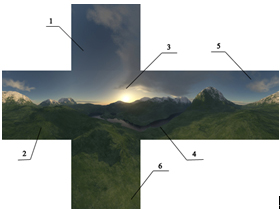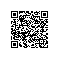# Silverlight C# 游戏开发：L9 天空盒1. public class Lesson09 : Lesson04
2. {
3.     public Lesson09()
4.     {
5.     }1. public class Lesson09 : Lesson04
2. {
3.     public Lesson09()
4.     {
5.         //创建天空盒
6.         Skybox skybox = new Skybox();
7.         //天空盒6个方向的指定贴图
14.         //激活天空盒，如果没有激活则不会显示出来
15.         skybox.IsEnabled = true;
16.         //指定
17.         game.Skybox = skybox;
18.     }
19.     double _sin = 0;
20.     //重写Lesson04中的Timer动画
21.     protected override void _dispatchertimer_Tick(object sender, EventArgs e)
22.     {
23.         //首先执行原来的
24.         base._dispatchertimer_Tick(sender, e);
25.         //判断是否存在摄像机
26.         if (game.Camera == null)
27.             return;
28.         //按照Sin曲线计算x，y，z的值，使之围绕浏览Camera的TargetPoint
29.         var x = System.Math.Cos(_sin) * 250;
30.         var y = System.Math.Sin(2*_sin) * 250;
31.         var z = System.Math.Sin(_sin) * 150;
32.         game.Camera.Position.X = x;
33.         game.Camera.Position.Y = y;
34.         game.Camera.Position.Z = z;
35.         //变化
36.         _sin += 0.016;
37.     }使用钉钉扫一扫加入圈子
+ 订阅